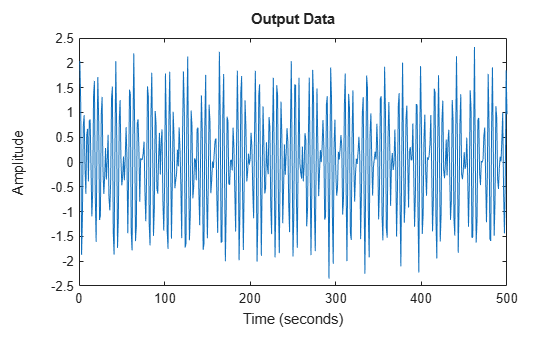# ivar

AR model estimation using instrumental variable method

## Syntax

``sys = ivar(data,na)``
``sys = ivar(data,na,nc)``
``sys = ivar(data,na,nc,max_size)``

## Description

example

````sys = ivar(data,na)` estimates an AR polynomial model, `sys`, using the instrumental variable method and the time series data `data`. `na` specifies the order of the A polynomial.An AR model is represented by the equation:$A\left(q\right)y\left(t\right)=e\left(t\right)$In the above model, e(t) is an arbitrary process, assumed to be a moving average process of order `nc`, and possibly time varying. The function assumes that `nc` is equal to `na`. Instruments are chosen as appropriately filtered outputs, delayed `nc` steps.```
````sys = ivar(data,na,nc)` specifies the value of the moving average process order `nc` independently.```
````sys = ivar(data,na,nc,max_size)` specifies the maximum size of matrices formed during estimation.```

## Examples

collapse all

Construct output data by combining sinusoidal signals and noise.

```y = iddata(sin([1:500]'*1.2) + sin([1:500]'*1.5) + 0.2*randn(500,1),[]); plot(y)```Estimate fourth-order models using the IV method (`ivar`) and the forward-backward least-squares method (`ar`).

```sysiv = ivar(y,4); sysls = ar(y,4);```

Compare the spectra of the two models.

```spectrum(sysiv,sysls) legend('ivar','ar')```The model estimated with `ivar` exhibits the two distinct spectral peaks. The model estimated with `ar` exhibits only one peak.

## Input Arguments

collapse all

Time-series data, specified as an `iddata` object that contains a single output channel and an empty input channel.

Order of the A polynomial, specified as a positive integer.

Order of the moving average process that represents e(t), specified as a positive integer.

Maximum matrix size for any matrix formed by the algorithm for estimation, specified as a positive integer. Specify `max_size` as a reasonably large value.

## Output Arguments

collapse all

Identified polynomial model, returned as a discrete-time `idpoly` model object.

Information about the estimation results and options used is stored in the `Report` property of the model. `Report` has the following fields.

Report FieldDescription
`Status`

Summary of the model status, which indicates whether the model was created by construction or obtained by estimation.

`Method`

Estimation command used.

`InitialCondition`

Handling of initial conditions during model estimation, returned as one of the following values:

• `'zero'` — The initial conditions were set to zero.

• `'estimate'` — The initial conditions were treated as independent estimation parameters.

This field is especially useful to view how the initial conditions were handled when the `InitialCondition` option in the estimation option set is `'auto'`.

`Fit`

Quantitative assessment of the estimation, returned as a structure. See Loss Function and Model Quality Metrics for more information on these quality metrics. The structure has the following fields:

FieldDescription
`FitPercent`

Normalized root mean squared error (NRMSE) measure of how well the response of the model fits the estimation data, expressed as the percentage fitpercent = 100(1-NRMSE).

`LossFcn`

Value of the loss function when the estimation completes.

`MSE`

Mean squared error (MSE) measure of how well the response of the model fits the estimation data.

`FPE`

Final prediction error for the model.

`AIC`

Raw Akaike Information Criteria (AIC) measure of model quality.

`AICc`

Small-sample-size corrected AIC.

`nAIC`

Normalized AIC.

`BIC`

Bayesian Information Criteria (BIC).

`Parameters`

Estimated values of model parameters.

`DataUsed`

Attributes of the data used for estimation, returned as a structure with the following fields.

FieldDescription
`Name`

Name of the data set.

`Type`

Data type.

`Length`

Number of data samples.

`Ts`

Sample time.

`InterSample`

Input intersample behavior, returned as one of the following values:

• `'zoh'` — Zero-order hold maintains a piecewise-constant input signal between samples.

• `'foh'` — First-order hold maintains a piecewise-linear input signal between samples.

• `'bl'` — Band-limited behavior specifies that the continuous-time input signal has zero power above the Nyquist frequency.

`InputOffset`

Offset removed from time-domain input data during estimation. For nonlinear models, it is `[]`.

`OutputOffset`

Offset removed from time-domain output data during estimation. For nonlinear models, it is `[]`.

For more information on using `Report`, see Estimation Report.

 Stoica, P., T. Soderstrom, and B. Friedlander. Optimal Instrumental Variable Estimates of the AR Parameters of an ARMA Process. IEEE Transactions on Automatic Control 30, no. 11 (November 1985): 1066–74, https://doi.org/10.1109/TAC.1985.1103839.